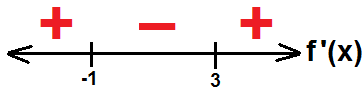# The Second Derivative

2 teachers like this lesson
Print Lesson

## Objective

SWBAT find inflection points and identify concavity.

#### Big Idea

Yes, the derivative of the derivative is a real thing and we will use it to further describe functions.

## Launch and Explore

15 minutes

Today's lesson will build upon what we already know as we look at the second derivative. Students may naturally wonder what the significance of taking the derivative of the derivative is, and we will explore that today.

I start by giving students this worksheet that includes a function, its derivative, and its second derivative. As a refresher, I have students recap what we learned about derivative graphs by looking at f(x) and identifying the critical points and matching them up to the corresponding points on the graph of f'(x). We also recap what we know about the original function if the first derivative is positive or negative.

Next, I explain that we can do the same process again to take the derivative of the derivative. Students may become confused and feel as if this is some type of mathematical inception, but I explain that this is called the second derivative and that it has some special properties.

To build some conceptual understanding of the second derivative, we look at the critical points of the first derivative and notice that those are the zeros of the second derivative. I explain that these points are called inflection points and that they have some significance on the original function. In the video below I explain my next teaching moves to give students some time to brainstorm the importance of inflection points.

## Share

15 minutes

After students have had time to brainstorm about the importance of the inflection points, we will share out our suggestions. Here are some thoughts that my students usually come up with:

• The inflection point is where the steepest slope will occur
• The inflection points split up the graph into parabolas
• The parabolas that the graph is split up into switch from pointing up to pointing down

These are all great suggestions and have some definite ties to concavity. If no one says it outright, at this point I will provide a hint to draw in tangent lines in the three portions of the graph to see if they can notice that the tangent lines switch sides. Once they notice that I will have them draw a diagram like this on their worksheet to summarize the importance of the inflection points.

Next, we will write down the definitions of inflection points, upward concavity, and downward concavity to summarize these main points.

## Extend

20 minutes

Now that we understand concavity and inflection points, we will put it to the test by trying to identify characteristics of a function algebraically without using a graph. Question #2 on the worksheet asks students to find out a lot about a given function. My main intent is that I want students to use sign diagrams to test intervals of the first and second derivative to find out about the original function.

I start by asking students to answer questions #2a with their table. I hope that students algebraically set the first and second derivative to zero to find these values. If they use a graphical approach I ask how they could do it without a graph.

For question #2b, I ask questions until students realize that in order to find out where the graph is increasing, we must find out where the first derivative is positive. I know that we could just graph the function and find these intervals, but I want them to see the connection to derivatives. To find out where the first derivative is positive or negative, I introduce a sign diagram (as shown below) and demonstrate how we can test each interval to see the value of f'(x).Once students understand the concept of a sign diagram, I have them create one for the second derivative to find the intervals of upward and downward concavity. I also have them finish the rest of the parts for question #2.

We covered a lot today, so I want to make sure that students can keep all of this organized in their mind. To finish the lesson, I ask the following questions (with answers) to summarize everything we learned about derivatives and their relation to the original function.

1. How do we find critical points of a function? Find where the first derivative is equal to zero or undefined.
2. How do we find inflection points of a function? Find where the second derivative is equal to zero.
3. How do we find where the original function is increasing? Find where the first derivative is positive.
4. How do we find where the original function has downward concavity? Find where the second derivative is negative.

Finally, I give this homework assignment to give some practice with the second derivative.